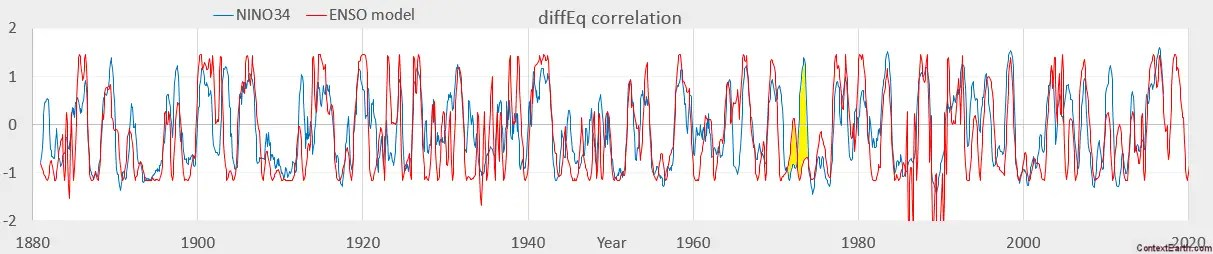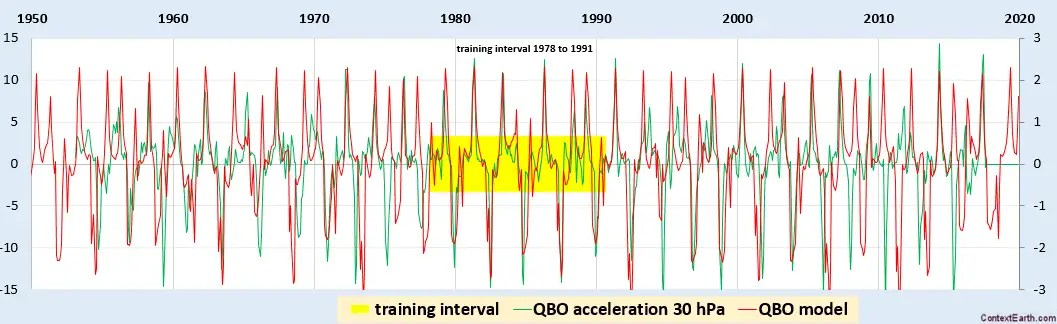# Approximating the ENSO Forcing Potential

From the last post, we tried to estimate the lunar tidal forcing potential from the fitted harmonics of the ENSO model. Two observations resulted from that exercise: (1) the possibility of over-fitting to the expanded Taylor series, and (2) the potential of fitting to the ENSO data directly from the inverse power law.

The Taylor’s series of the forcing potential is a power-law polynomial corresponding to the lunar harmonic terms. The chief characteristic of the polynomial is the alternating sign for each successive power (see here), which has implications for convergence under certain regimes. What happens with the alternating sign is that each of the added harmonics will highly compensate the previous underlying harmonics, giving the impression that pulling one signal out will scramble the fit. This is conceptually no different than eliminating any one term from a sine or cosine Taylor’s series, which are also all compensating with alternating sign.

The specific conditions that we need to be concerned with respect to series convergence is when r (perturbations to the lunar orbit) is a substantial fraction of R (distance from earth to moon) :$F(r) = frac{1}{(R+r)^3}$

Because we need to keep those terms for high precision modeling, we also need to be wary of possible over-fitting of these terms — as the solver does not realize that the values for those terms have the constraint that they derive from the original Taylor’s series. It’s not really a problem for conventional tidal analysis, as the signals are so clean, but for the noisy ENSO time-series, this is an issue.

Of course the solution to this predicament is not to do the Taylor series harmonic fitting at all, but leave it in the form of the inverse power law. That makes a lot of sense — and the only reason for not doing this until now is probably due to the inertia of conventional wisdom, in that it wasn’t necessary for tidal analysis where harmonics work adequately.

So this alternate and more fundamental formulation is what we show here.

The starting ansatz we apply is to create two potentials with varying amounts of draconic and anomalistic factors in each denominator:$F(r) = frac{1}{(R+a_0(t)+d_0(t))^3} - frac{1}{(R+a_1(t)+d_1(t))^3}$

Each of the lunar factors is a combination of the lunar monthly and fortnightly terms, so that we have only four degrees of freedom (DOF) in terms of amplitude and only two in phase (since the monthly and fortnightly cycles share the same phase for each lunar mode).

Given the fact that conventional tidal analysis can contain hundreds of harmonic factors,this obviously won’t work unless we get the terms as accurately and precisely represented as we can, including the large variations in the anomalistic cycle and the smaller but still significant variations in the draconic cycle.For example, the variation in the apogee and perigee distance in the anomalistic cycle is critical. Previously, we included the variation in the velocity of the cycle, but the variation in the distance over the elliptical cycle is also significant. This known profile can either be constrained as a configuration parameter or left to automatically converge as this fit revealed:Paying attention to this detail to a meticulous and almost ridiculous degree is what pays off in generating an excellent fit.Lunar monthly and fortnightly draconic and monthly and fortnightly anomalistic forcing. Mismatch at ~1974

The two potentials suggested as the ansatz ultimately split into two terms that feature predominantly anomalistic factors in one potential and exclusively draconic factors in the other potential.$F(r) = frac{1}{(R+a(t)+ epsilon d(t))^3} - frac{1}{(R+d(t))^3}$

This simpler formulation should result in less over-fitting in cross-validation training intervals. The figure below uses only data to 1980 and the solver has complete freedom to readjust the parameters, yet still correlates to the extrapolated post-1980 data.Compared to the number of harmonic factors used in previous modeling results, this canonical tidal potential model has a much reduced complexity. The fewer the number of adjustable parameters, the less the model can over-fit to noise within the data series.

Is we introduce more constraints, then we further reduce the risk of over-fitting and potential divergence for predictions. So if we add the draconic inclination variation as shown below, this should help constrain and align the draconic waveform.For this extra constraint we consider the following formulation$F(r) = sqrt{ (frac{1}{(R+a(t))^3} -1)^2 + (frac{1}{(R+d(t))^3}-1)^2 }$

This results in the following aggressive cross-validating fits:Probably a good time to comment on the status of the analysis. The journey to modeling ENSO and QBO has been circuitous and then essentially doubled back to the most basic kind of forcing and the simplest toy differential equations.

The ENSO behavior is modeled as two lunar tidal signals and an annual forcing impulse applied to a delay differential equation of 1-year delay. Could have started with this premise from day one, but nothing in the research literature indicated lunar forcing had any effect on ENSO.

Same goes for QBO, except that it is essentially a single lunar tidal signal and a bi-annual seasonal forcing signal — one impulse per nodal crossing. Because we remove a set of degrees-of-freedom due to the anomalistic tide and keep the draconic, the interval for cross-validation can be much reduced.And over the entire interval the fit looks equivalent, but note that a projection from 2016-2017 appears to confirm the curious QBO anomaly of last year. It now appears to be back in sync according to the model.The climate-change-denier and early QBO-researcher Richard Lindzen had considered lunar forcing for QBO but apparently couldn’t find any correlation, and that’s why no one followed up in that regard. A lack of detailed metrology-level modeling on Lindzen’s part likely convinced ensuing scientists not to continue to check for a lunar cause.

Because of the clarity of the single lunar mechanism for QBO, I definitely have not spent as much time on that data set. In contrast, the ENSO puzzle has been much more involved due to the extra DOF complexity. Looking back on my first blog post with respect to the ENSO topic was dated early 2014, so it’s been almost 4 years of spare-time effort. And even though this was anticipated to be a software coding project, the model is simple enough to express on a spreadsheet without the need for any macros or scripts except for a standard Solver plugin. It’s essentially a little more complex than a basic tidal analysis program, and perhaps even simpler than that considering that the harmonics are subsumed in a tidal potential formulation.

Like Lindzen, the AGW-skeptic and ENSO-researcher Anastosios Tsonis gave up much too early in the analysis when he proposed a chaotic regime for the ENSO behavior. He took Lindzen’s lead and simply asserted that the mode was chaotic. In reality, chaos can only be assumed as a mechanism when every other mechanism has been eliminated. And it’s clear with the lunar forcing mechanism, that chaos should be re-assigned as a backup candidate.

Apologies for this bit of subjectivity, but I have to toss these rationalizations out there for target practice, since I will be presenting these results at next month’s AGU:

 GC41B-1022: Biennial-Aligned Lunisolar-Forcing of ENSO: Implications for Simplified Climate ModelsSure to get questions so will need to be able to answer the perennial question: What’s wrong with the consensus model? or Why shouldn’t we believe the consensus model?

“A complex system that works is invariably found to have evolved from a simple system that worked. A complex system designed from scratch never works and cannot be patched up to make it work. You have to start over, beginning with a working simple system.” — Gall’s Law

There really is no possible way that Lindzen’s complex model describes QBO, and neither should one start with a chaos model as espoused by Tsonis to describe ENSO. You really need to start with a simple model that explains the behavior, and work from there.

## Prologue:

About the title of this post, and the use of the word “approximate” — approximating a physical phenomenon with a math model shouldn’t be as controversial as it’s sometimes made out to be. Consider the way that George Box’s phrase of “All models are wrong, some are useful” has often been misinterpreted. Although Box apparently used this phrase in several contexts, the one quoted here is appropriate:from: Response Surfaces, Mixtures, and Ridge Analyses, George E. P. Box, Norman R. Draper, (2007) Wiley

This passage in fact has nothing to do with the applicability of a physical model, but whether an approximate mathematical model is sufficient to represent enough accuracy and/or precision to the more detailed model. Box states right there that the polynomial that he is evaluating is an approximation to a more refined view and he is explaining how to benefit from the approximation and to understand its mathematical limitations in terms of any systematic error it will introduce.

So, to place this into the context of this post and the previous post, we had been working on a polynomial (i.e. Taylor’s series) approximation to the better mathematical formulation of Newton’s inverse power law for gravitational forcing to ENSO. So although the polynomial approximation allowed significant process to be made, it was still only an approximation — and thus “wrong” although “useful” according to Box’s terminology. And from this post, one can be convinced that the non-approximated form of lunar forcing does work much better, and is much simplified in comparison to the polynomial series approximation.

The misinterpretation of Box’s quote has been especially rife in the denial-sphere, whereby any physical model is deemed as “wrong”, instead of a continuous refinement to obtaining the “right” answer.

## 5 thoughts on “Approximating the ENSO Forcing Potential”

1.admin

Another article on the QBO anomaly

http://onlinelibrary.wiley.com/doi/10.1002/2017GL075576/full

C. A. Barton and J. P. McCormack, “Origin of the 2016 QBO Disruption and Its Relationship to Extreme El Niño Events,” Geophys. Res. Lett.

“The regularity of the QBO since its discovery has underscored its high predictability and promoted its utility for skillful long-range prediction”

The issue is not that everyone realizes that QBO is regular (in contrast to ENSO, which is not obvious to the eye), but that they do not have the underlying mechanism for the regularity understood.

Like

2.admin

This is a neat analysis paper deriving lunar cycles
CHALUB, Fabio A.C.C.. The Saros cycle: obtaining eclipse periodicity from Newton’s laws. Rev. Bras. Ensino Fís. 2009, vol.31, n.1 . http://dx.doi.org/10.1590/S1806-11172009000100003.

Also
Long Period Tidal Force Variations and Regularities in Orbital Motion of the Earth-Moon Binary Planet System

Like

3.admin

Minimal filtering (triangle = 0.5 of month + 0.25 each adjacent month )

This comprises only two fundamental sinusoidal waves to generate the model fit, transformed by a seasonal impulse and Laplace’s tidal equation for the equator.

Given the fact that the ENSO signal is considered chaotic, no one could expect that 2 sinusoids could do the job. How could it? Well, that’s the characteristic of the natural world. It can easily hide the order and regularity via the most subtle of response functions.

Like